# A.1 Transport under a Velocity-Dependent Acceleration

This section is devoted to describe the derivation of the equations of motion (5.3)-(5.12) for a droplet experiencing a force in the direction of its motion such as gravity as well as forces working against its motion such as the thermophoretic force and the velocity-dependent Stokes force. For now, it is assumed that there is no influence from the electric force on the droplet motion. The first step is to equate all forces acting on the droplet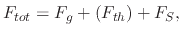(208)

where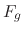is the force due to gravity,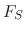is the Stokes force, and the thermophoretic force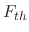is in brackets, because it is only included, when the droplet reaches the heat zone, and at that point, the radius must be modified according to its evaporation. However, for the following derivations, a constant radius will be assumed. Therefore, when the thermophoretic force is to be included, a smaller radiusis carried through the equations. The forces can be converted to droplet acceleration using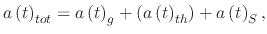(209)

where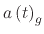,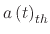, andare the droplet accelerations due to gravity, the thermophoretic force, and the Stokes force, respectively. Since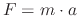, then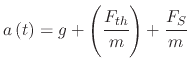(210)

and the droplet mass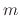is given by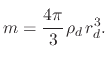(211)

The explicit equation for the droplet acceleration can be derived by substituting the relevant forces and the droplet massinto (A.3)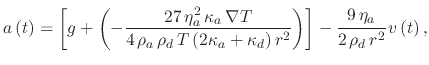(212)

where the meaning and values for the droplet and ambient parameters in (A.5) can be found in Table 6.1. In order to simplify the derivation, all constant values will be grouped together and only two constants will remain in the form of the independent constant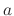and constant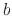, which relates the velocity dependence of the acceleration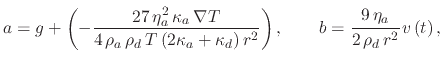(213)

allowing for (A.5) to be rewritten as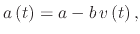(214)

The solution to (A.7) is found by first noting that it is a differential equation(215)

which can be re-written in the form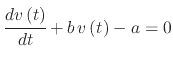(216)

and converted into Euler's characteristic equation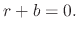(217)

This characteristic equation only has one root,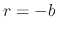, meaning the velocity can be solved by writing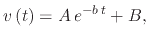(218)

where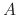and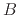are constants. In order to findand, two assumptions must be made: the initial velocity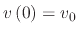is known and at some time, the velocity will reach a terminal velocity due to the equivalent and opposite forces acting on the droplet, meaning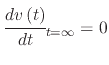. Therefore, whenapproaches infinity,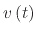from (A.11) approaches, meaning thatis the terminal velocity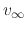. This can be substituted into (A.7) to obtain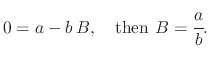(219)

Now thatand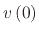are known, they can be substituted into (A.11) to find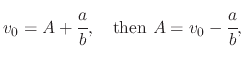(220)

giving the final description for the velocity of the droplet at any time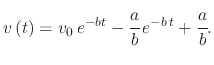(221)

In order to find the displacement of the droplet under the given forces, (A.14) can be integrated from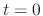, when the initial displacement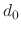is set to 0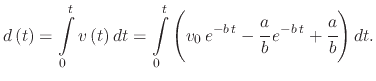(222)

resulting in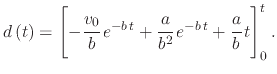(223)

The displacement is then given by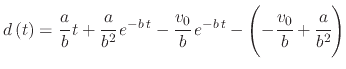(224)

or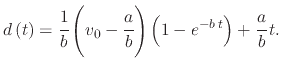(225)

The timeis needed in order to calculate the droplet displacement. As the droplet travels through the ambient, it is much easier to calculate the time required for the droplet to reach the wafer followed by the calculation of the radial direction displacement. This would make simulations much quicker than a discretization of the timein order to follow the droplet trajectory. The time is found by inverting (A.18)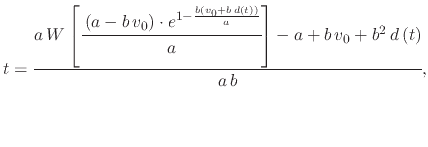(226)

where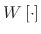is the Lambert-W function which is solved iteratively and is defined by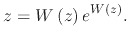(227)

L. Filipovic: Topography Simulation of Novel Processing Techniques